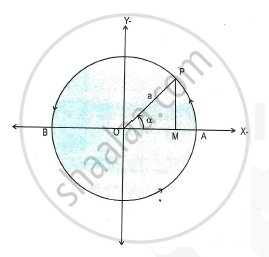# Define Linear S.H.M. - Physics

Define linear S.H.M.

#### Solution

Linear S.H.M. is defined as the linear periodic motion of a body in which the restoring force (or acceleration) is always directed towards its mean position and its magnitude is directly proportional to the displacement from the mean position.
Consider a particle ‘P’ moving along the circumference of a circle of radius 'a' and centre O, with uniform angular speed of 'ω' in anticlockwise direction as shown.
Particle P along circumference of the circle has its projection particle on diameter AB at point M.Concept: Differential Equation of Linear S.H.M.
Is there an error in this question or solution?
2015-2016 (March)

Share